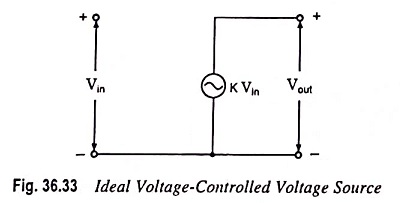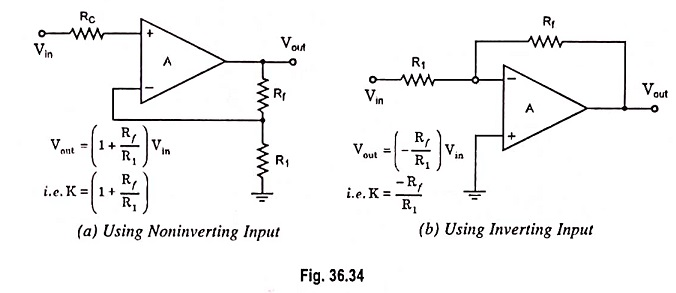## Voltage Controlled Voltage Source (VCVS) Circuit:

An ideal form of a voltage source, whose output voltage Vout is controlled by an input voltage Vin, is given in Fig. 36.33.The output voltage is seen to be dependent on the input voltage (times a scale factor K). Such a circuit can be built by connecting an op-amp either in noninverting mode or in inverting mode, as shown in Fig. 36.34 (a) and 36.34 (b) respectively.The circuit shown in Fig. 36.34 (b) can be used as a multiplier or divider. For Rf > R1, the circuit acts as a multiplier and for Rf < R1 it acts as a divider.

Scroll to Top